# Sliding motion along an inclined plane

This article discusses a scenario/arrangement whose statics/dynamics/kinematics can be understood using the ideas of classical mechanics.
View other mechanics scenarios

The scenario here is a dry block (with a stable surface of contact) on a dry fixed inclined plane, with$\theta$ being the angle of inclination with the horizontal axis.

The extremes are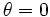$\theta = 0$ (whence, the plane is horizontal) and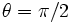$\theta = \pi/2$ (whence, the plane is vertical).

## Basic components of force diagram

A good way of understanding the force diagram is using the coordinate axes as the axis along the inclined plane and normal to the inclined plane. For simplicity, we will assume a two-dimensional situation, with no forces acting along the horizontal axis that is part of the inclined plane (in our pictorial representation, this no action axis is the axis perpendicular to the plane).

### Component perpendicular to the inclined plane

In this case, assuming a stable surface of contact, and that the inclined plane does not break under the weight of the block, and no other external forces, we get the following equation from Newton's first law of motion applied to the axis perpendicular to the inclined plane: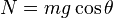$\! N = mg \cos \theta$

where$N$ is the normal force between the block and the inclined plane,$m$ is the mass of the block, and$g$ is the acceleration due to gravity.$N$ acts inward on the inclined plane and outward on the block. Some observations:

Value/change in value of$\theta$ Value of$N$ Comments$\theta = 0$ (horizontal plane)$N = mg$ The normal force exerted on a horizontal surface equals the mass times the acceleration due to gravity, which we customarily call the weight.$\theta = \pi/2$ (vertical plane)$N = 0$ The block and the inclined plane are barely in contact and hardly pressed together.$\theta$ increases from$0$ to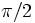$\pi/2$$N$ reduces from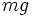$mg$ to$0$. The derivative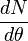$\frac{dN}{d\theta}$ is$-mg\sin \theta$ The force pressing the block and the inclined plane reduces as the slope of the incline increases.

For simplicity, we ignore the cases$\theta = 0$ and$\theta = \pi/2$ unless specifically dealing with them.

### Component along (down) the inclined plane

For the axis down the inclined plane, the gravitational force component is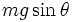$mg\sin \theta$. The magnitude and direction of the friction force are not clear. We have three cases:

Case Convention on the sign of acceleration$a$ Equation Simplified, after combining with$\! N = mg \cos \theta$
Block sliding down the inclined plane measured positive down the inclined plane, hence a positive number$\! ma = mg \sin \theta - \mu_kN$$\! a = g (\sin \theta - \mu_k\cos \theta) = g \cos \theta (\tan \theta - \mu_k)$
Block sliding up the inclined plane measured positive down the inclined plane (note that acceleration opposes direction of motion, leading to retardation)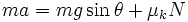$\! ma = mg \sin \theta + \mu_kN$$\! a = g (\sin \theta + \mu_k \cos \theta) = g \cos \theta (\tan \theta + \mu_k)$
Block stationary no acceleration, so no sign convention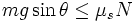$\! mg \sin \theta \le \mu_sN$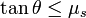$\! \tan \theta \le \mu_s$

## Behavior for a block initially placed at rest

### Statics and dynamics

If the block is initially placed gently at rest, we have the following cases:

Case What happens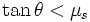$\tan \theta < \mu_s$, or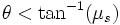$\theta < \tan^{-1}(\mu_s)$ The block does not start sliding down, and instead stays stable where it is. The magnitude of static friction is$mg \sin \theta$, and its direction is upward along the inclined plane, precisely balancing and hence canceling the component of gravitational force along the inclined plane.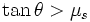$\tan \theta > \mu_s$ or$\theta > \tan^{-1}(\mu_s)$ The block starts sliding down, and the downward acceleration is given by$a = g(\sin \theta - \mu_k \cos \theta) = g \cos \theta (\tan \theta - \mu_k)$.

The angle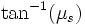$\tan^{-1}(\mu_s)$ is termed the angle of repose, since this is the largest angle at which the block does not start sliding down.

### Kinematics

The kinematic evolution in the second case is given as follows, if we set$t = 0$ as the time when the block is placed, we have the following (here, the row variable is written in terms of the column variable):$\! v$$\! t$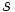$\! s$ vertical displacement (call$\!h$) horizontal displacement (call$\! x$)$\! v$$\! v$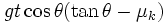$\! gt\cos \theta(\tan \theta - \mu_k)$$\! \sqrt{2sg\cos \theta (\tan \theta - \mu_k)}$$\! \sqrt{2hg(1 - \mu_k \cot \theta)}$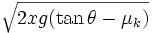$\! \sqrt{2xg(\tan \theta - \mu_k)}$$\! s$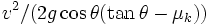$\! v^2/(2g\cos \theta (\tan \theta - \mu_k))$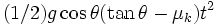$\! (1/2)g \cos \theta (\tan \theta - \mu_k)t^2$$\! s$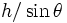$\! h/\sin \theta$$\! x/\cos \theta$$\! h$$\! v^2 \tan \theta/(2g(\tan \theta - \mu_k))$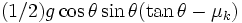$\! (1/2) g \cos \theta \sin \theta (\tan \theta - \mu_k)$$\! s \sin \theta$$\! h$$\! x \tan \theta$$\! x$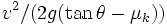$\! v^2/(2g (\tan \theta - \mu_k))$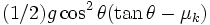$\! (1/2)g \cos^2 \theta (\tan \theta - \mu_k)$$\! s \cos \theta$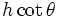$\! h \cot \theta$$\! x$

## Given an initial speed downward

### Statics and dynamics

If the block is given an initial downward speed, the acceleration is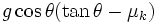$g \cos \theta (\tan \theta - \mu_k)$ downward, and its long-term behavior is determined by whether$\tan \theta < \mu_k$ or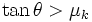$\tan \theta > \mu_k$:

• If$\tan \theta < \mu_k$, then the block's speed reduces with time. The magnitude of retardation is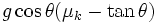$g \cos \theta (\mu_k - \tan \theta)$. If the incline is long enough, this retardation continues until the block reaches a speed of zero, at which point it comes to rest and thence stays at rest.
• If$\tan \theta > \mu_k$, then the block's speed increases with time. The magnitude of acceleration is$g \cos \theta (\tan \theta - \mu_k)$. The block thus does not come to a stop and keeps going faster as it goes down the incline.

### Kinematics

Fill this in later

### Energy changes

Fill this in later

## Given an initial speed upward

### Statics and dynamics

If the block is given an initial upward speed, the acceleration is$g \cos \theta (\tan \theta + \mu_k)$ downward, i.e., the retardation is$g \cos \theta(\tan \theta + \mu_k)$. We again consider two cases:

Case What happens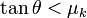$\! \tan \theta < \mu_k$ or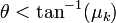$\! \theta < \tan^{-1}(\mu_k)$ The block's speed reduces with time as it rises. If the incline is long enough, the block eventually comes to a halt and stays still after that point.$\! \tan \theta$ is between$\! \mu_k$ and$\! \mu_s$ The behavior is somewhat indeterminate, in the sense that whether the block starts sliding down after stopping its upward slide is unclear.$\! \tan \theta > \mu_s$ The block's speed reduces with time as it rises, until it comes to a halt, after which it starts sliding downward, just as in the case of a block initially placed at rest.

### Kinematics

Suppose the initial upward speed is$u$. Because of our downward positive convention, we will measure the velocity as$-u$. Then, we have:

Quantity Value
Time taken to reach highest point$\! t = \frac{u}{g \cos \theta (\tan \theta + \mu_k)}$
Displacement to highest point achieved (along incline)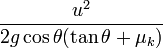$\frac{u^2}{2g \cos \theta (\tan \theta + \mu_k)}$
Vertical height to highest point achieved$\frac{u^2 \tan \theta}{2g (\tan \theta + \mu_k)}$
Horizontal displacement to highest point achieved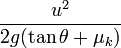$\frac{u^2}{2g(\tan \theta + \mu_k)}$

In case that$\tan \theta > \mu_s$, the block is expected to slide back down and return to the original position. The kinematics of the downward motion are the same as those for a block initially placed at rest. Two important values are given below:

Quantity Value
Time taken on return journey$\! \frac{u}{g \cos \theta}{\tan^2 \theta - \mu_k^2}$
Speed at instant of return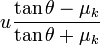$\! u \frac{\tan \theta - \mu_k}{\tan \theta + \mu_k}$

### Energy changes

Fill this in later